# RD Sharma Class 8 Solutions Chapter 6 Algebraic Expressions and Identities Ex 6.6

In this chapter, we provide RD Sharma Class 8 Solutions Chapter 6 Algebraic Expressions and Identities Ex 6.6 for English medium students, Which will very helpful for every student in their exams. Students can download the latest RD Sharma Class 8 Solutions Chapter 6 Algebraic Expressions and Identities Ex 6.6 Maths pdf, free RD Sharma Class 8 Solutions Chapter 6 Algebraic Expressions and Identities Ex 6.6 Maths book pdf download. Now you will get step by step solution to each question.

### RD Sharma Solutions for Class 8 Chapter 6 Algebraic Expressions and Identities Ex 6.6Download PDF

Question 1.
Write the following squares of bionomials as trinomials :Solution:
Using the formulas
(a + b)2 = a2 + 2ab + band (a – b)2 = a2 – 2ab + b2(i) (a + 2)2 = (a)2 + 2 x a x 2 + (2)2
{(a + b)2 = a2 + 2ab + b2}
= a2 + 4a + 4
(ii) (8a + 3b)2 = (8a)2 + 2 x 8a * 3b + (3b)= 642 + 48ab + 9 b2
(iii) (2m+ 1)2 = (2m)2 + 2 x 2m x1 + (1)2
= 4m2 + 4m + 1Question 2.
Find the product of the following binomials :Solution:Question 3.
Using the formula for squaring a binomial, evaluate the following :

(i) (102)2
(ii) (99)2
(iii) (1001)2

(iv) (999)2
(v) (703)
2
Solution:
(i)
(102)2 = (100 + 2)2
= (100)2 + 2 x 100 x 2 + (2)2
{(a + b)2 = a2 + 2ab + b2}
= 10000 + 400 + 4 = 10404
(ii) (99)2 = (100 – 1)2
= (100)2 – 2 x 100 X 1 +(1)2
{(a – b)2 = a2 – 2ab + b2}
= 10000 -200+1
= 10001 -200 =9801
(iii) (1001 )2 = (1000 + 1)2
{(a + b)2 = a2 + 2ab + b2}
= (1000)2 + 2 x 1000 x 1 + (1)2
= 1000000 + 2000 + 1 = 1002001
(iv) (999)2 = (1000 – 1)2
{(a – b)2 = a2 – 2ab + b2}
= (1000)2 – 2 x 1000 x 1 + (1)2
= 1000000 – 2000 + 1
= 1000001 -2000 = 998001

Question 4.
Simplify the following using the formula:

(a – b) (a + b) = a2 – b2 :
(i) (82)2 (18)2
(ii) (467)2 (33)2
(iii) (79)2 (69)2
(iv) 197 x 203
(v) 113 x 87
(vi) 95 x 105
(vii) 1.8 x 2.2
(viii) 9.8 x 10.2
Solution:
(i)
(82)2 – (18)2 = (82 + 18) (82 – 18)
{(a + b)(a- b) = a2 – b2} = 100 x 64 = 6400
(ii) (467)2 – (33)2 = (467 + 33) (467 – 33)
= 500 x 434 = 217000
(ii) (79)2 – (69)2 = (79 + 69) (79 – 69)
148 x 10= 1480
(iv) 197 x 203 = (200 – 3) (200 + 3)
= (200)2 – (3)2
= 40000-9 = 39991
(v) 113 x 87 = (100 + 13) (100- 13)
= (100)2 – (13)2
= 10000- 169 = 9831
(vi) 95 x 105 = (100 – 5) (100 + 5)
= (100)2 – (5)2
= 10000 – 25 = 9975
(vii) 8 x 2.2 = (2.0 – 0.2) (2.0 + 0.2)
= (2.0)2 – (0.2)2
= 4.00 – 0.04 = 3.96
(viii)9.8 x 10.2 = (10.0 – 0.2) (10.0 + 0.2)
(10.0)2 – (0.2)2
= 100.00 – 0.04 = 99.96

Question 5.
Simplify the following using the identities :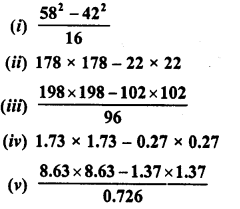Solution:Question 6.
Find the value of x, if
(i)  4x = (52)2 – (48)2
(ii) 14x = (47)2 – (33)2
(iii)  5x = (50)2 – (40)2
Solution:
(i)
4x = (52)2 – (48)2
⇒ 4x = (52 + 48) (52 – 48)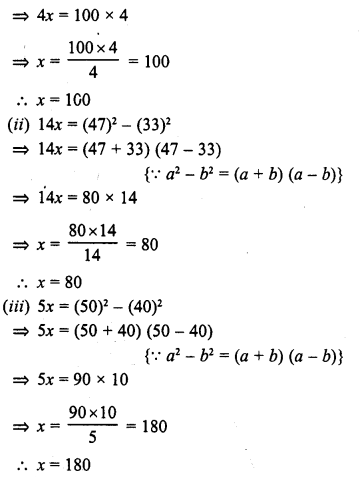Question 7.
If x + 1x= 20, find the value of x2+ 1×2

Solution:Question 8.
If x – 1x = 3, find the values of x2 + 1×2 and x4 + 1×4

Solution:Question 9.
If x2 – 1×2= 18, find the values of x+ 1x  and x– 1x
Solution:Question 10.
Ifx+y = 4 and xy = 2, find the value of x2+y2.

Solution:
x + y = 4
Squaring on both sides,
(x + y)2 = (4)2
⇒ x2 +y2 + 2xy = 16
⇒ x2+y2 + 2 x 2 = 16                       (∵ xy = 2)
⇒ x2 + y2 + 4 = 16
⇒ x2+y2 = 16 – 4= 12           ‘
∴ x2+y2 = 12

Question 11.
If x-y = 7 and xy = 9, find the value of x2+y2.
Solution:
x-y = 7
Squaring on both sides,
(x-y)2 = (7)2
⇒ x2+y2-2xy = 49
⇒ x2 + y2 – 2 x 9 = 49                    (∵ xy = 9)
⇒ x2 +y2 – 18 = 49
⇒ x2 + y2 = 49 + 18 = 67
∴ x2+y2 = 67

Question 12.
If 3x + 5y = 11 and xy = 2, find the value of 9x2 + 25y2
Solution:
3 x + 5y = 11, xy = 2
Squaring on both sides,
(3x + 5y)2 = (11)2
⇒ (3x)2 + (5y)2 + 2 x 3x x 5y = 121
⇒ 9x2 + 25y2 + 30 x 7 = 121
⇒ 9x2 + 25y2+ 30 x 2 = 121           (∵ xy = 2)
⇒ 9x2 + 25y2 + 60 = 121
⇒ 9x2 + 25y2 = 121 – 60 = 61
∴ 9x2 + 25y2 = 61

Question 13.
Find the values of the following expressions :

(i)16x2 + 24x + 9, when X’ = 745
(ii) 64x2 + 81y2 + 144xy when x = 11 and y = 43
(iii) 81x2 + 16y2-72xy, whenx= 23 andy= 34
Solution: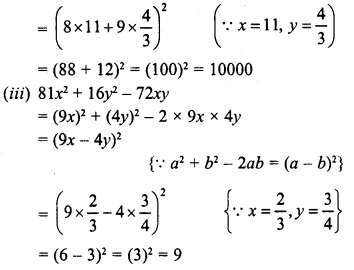Question 14.
If x + 1x = 9, find the values of x+ 1×4.
Solution: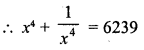Question 15.
If x + 1x = 12, find the values of x–  1x.
Solution:Question 16.
If 2x + 3y = 14 and 2x – 3y = 2, find the value of xy.
Solution:
2x + 3y = 14, 2x – 3y= 2
We know that
(a + b)2 – (a – b)2 = 4ab
∴ (2x + 3y)2 – (2x – 3y)2 = 4 x 2x x 3y = 24xy
⇒ (14)2 – (2)2 = 24xy
⇒ 24xj= 196-4= 192
⇒ xy = 19224 = 8
∴  xy = 8

Question 17.
If x2 + y2 = 29 and xy = 2, find the value of
(i) x+y
(ii) x-y
(iii) x4 +y4
Solution:
x2 + y2 = 29, xy = 2
(i) (x + y)2 = x2 + y2 + 2xy
= 29 + 2×2 = 29+ 4 = 33
∴  x + y= ±√33
(ii) (x – y)2 = x2 + y2 – 2xy
= 29- 2×2 = 29- 4 = 25
∴ x-y= ±√25= ±5
(iii) x2 + y2 = 29
Squaring on both sides,
(x2 + y2)2 = (29)2
⇒ (x2)2 + (y2)2 + 2x2y2 = 841
⇒ x4 +y + 2 (xy)2 = 841
⇒ x4 + y + 2 (2)2 = 841          (∵ xy = 2)
⇒ x4 + y + 2×4 = 841
⇒ x4 + y + 8 = 841
⇒ x4 + y = 841 – 8 = 833
∴ x4 +y = 833

Question 18.
What must be added to each of the following expressions to make it a whole square ?’
(i) 4x2 – 12x + 7
(ii) 4x2 – 20x + 20
Solution:
(i) 4x2 – 12x + 7 = (2x)2 – 2x 2x x 3 + 7
In order to complete the square,
we have to add  32 – 7 = 9 – 7 = 2
∴ (2x)2 – 2 x 2x x 3 + (3)2
= (2x-3)2
∴ Number to be added = 2
(ii) 4x2 – 20x + 20
⇒ (2x)2 – 2 x 2x x 5 + 20
In order to complete the square,
we have to add (5)2 – 20 = 25 – 20 = 5
∴ (2x)2 – 2 x 2x x 5 + (5)2
= (2x – 5)2
∴ Number to be added = 5

Question 19.
Simplify :
(i) (x-y) (x + y) (x2 + y2) (x4 + y4)
(ii) (2x – 1) (2x + 1) (4x2 + 1) (16x4 + 1)
(iii) (7m – 8m)2 + (7m + 8m)2
(iv) (2.5p -5q)2 – (1.5p – 2.5q)2
(v) (m2 – n2m)2 + 2m3n2

Solution:
(i) (x – y) (x + y) (x2 + y2) (x4 +y)
= (x2 – y2) (x2 + y) (x4 + y4)
= [(x2)2 – (y2)2] (x4+y4)
= (x4-y4) (x4+y4)
= (x4)2 – (y4)2 = x8 – y8
(ii) (2x – 1) (2x + 1) (4x2 + 1) (16x4 + 1)
= [(2x)2 – (1)2] (4x2 + 1) (16x4 + 1)
= (4x2 – 1) (4x2 + 1) (16x4 + 1)
= [(4x2)2-(1)2] (16x4+ 1)
= (16x4-1) (16x4+ 1)
= (16x4)2– (1)= 256x8 – 1
(iii) (7m – 8m)2 + (7m + 8n)2
= (7m)2 + (8n)2 – 2 x 7m x 8n + (7m)2 + (8n)2 + 2 x 7m x 8n
= 49m2 + 64m2 – 112mn + 49m2 + 64m2 + 112mn
= 98 m2 + 128n2
(iv) (2.5p – 1.5q)2 – (1.5p – 2.5q)2
= (2.5p)2 + (1.5q)2 – 2 x 2.5p x 1.5q
= [(1.5p)2 + (1.5q)2 – 2 x 1.5 p x 2.5q]
= (6.25p2 + 2.25q2 – 7.5 pq) – (2.25p2 + 6.25q2-7.5pq)
= 6.25p2 + 2.25q2 – 7.5pq – 2.25p2 – 6.25q+ 7.5pq
= 6.25p2 – 2.25p2 + 2.25g2 – 6.25q2
= 4.00P2 – 4.00q2
= 4p2 – 4q2 = 4 (p2 – q2)
(v) (m2 – n2m)2 + 2m3M2
= (m2)2 + (n2m)2 -2 x m2 x n2m + 2;m3m2
= m4 + n4m2 – 2m3n2 + 2m3n2
= m4 + n4m2 = m4 + m2n4

Question 20.
Show that :Solution: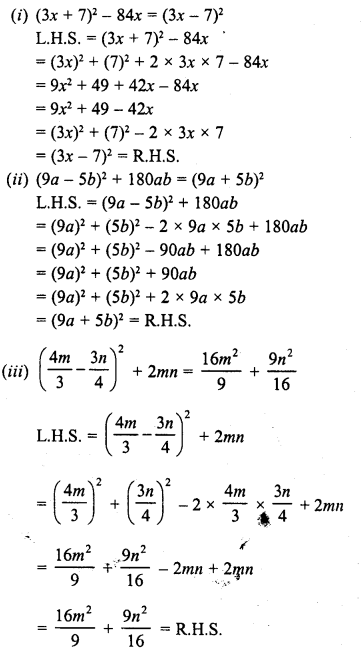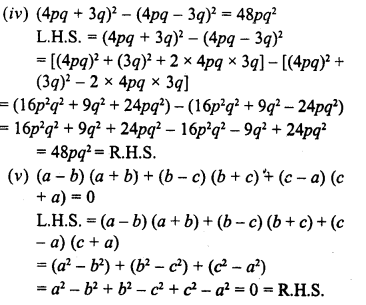All Chapter RD Sharma Solutions For Class 8 Maths

—————————————————————————–

All Subject NCERT Exemplar Problems Solutions For Class 8

All Subject NCERT Solutions For Class 8

*************************************************

I think you got complete solutions for this chapter. If You have any queries regarding this chapter, please comment on the below section our subject teacher will answer you. We tried our best to give complete solutions so you got good marks in your exam.

If these solutions have helped you, you can also share rdsharmasolutions.in to your friends.